# 3.3E: Exercises

$$\newcommand{\vecs}{\overset { \rightharpoonup} {\mathbf{#1}} }$$ $$\newcommand{\vecd}{\overset{-\!-\!\rightharpoonup}{\vphantom{a}\smash {#1}}}$$$$\newcommand{\id}{\mathrm{id}}$$ $$\newcommand{\Span}{\mathrm{span}}$$ $$\newcommand{\kernel}{\mathrm{null}\,}$$ $$\newcommand{\range}{\mathrm{range}\,}$$ $$\newcommand{\RealPart}{\mathrm{Re}}$$ $$\newcommand{\ImaginaryPart}{\mathrm{Im}}$$ $$\newcommand{\Argument}{\mathrm{Arg}}$$ $$\newcommand{\norm}{\| #1 \|}$$ $$\newcommand{\inner}{\langle #1, #2 \rangle}$$ $$\newcommand{\Span}{\mathrm{span}}$$ $$\newcommand{\id}{\mathrm{id}}$$ $$\newcommand{\Span}{\mathrm{span}}$$ $$\newcommand{\kernel}{\mathrm{null}\,}$$ $$\newcommand{\range}{\mathrm{range}\,}$$ $$\newcommand{\RealPart}{\mathrm{Re}}$$ $$\newcommand{\ImaginaryPart}{\mathrm{Im}}$$ $$\newcommand{\Argument}{\mathrm{Arg}}$$ $$\newcommand{\norm}{\| #1 \|}$$ $$\newcommand{\inner}{\langle #1, #2 \rangle}$$ $$\newcommand{\Span}{\mathrm{span}}$$$$\newcommand{\AA}{\unicode[.8,0]{x212B}}$$

# Section Exercises

## Verbal

1. Explain the difference between the coefficient of a power function and its degree.

Answer: The coefficient of the power function is the real number that is multiplied by the variable raised to a power. The degree is the highest power appearing in the function.

2. If a polynomial function is in factored form, what would be a good first step in order to determine the degree of the function?

3. In general, explain the end behavior of a power function with odd degree if the leading coefficient is positive.

Answer: As $$x$$ decreases without bound, so does $$f(x)$$. As $$x$$ increases without bound, so does $$f(x)$$.

4. What is the relationship between the degree of a polynomial function and the maximum number of turning points in its graph?

5. What can we conclude if, in general, the graph of a polynomial function exhibits the following end behavior? As x→−∞, $$f(x)→−∞$$ and as x→∞, $$f(x)→−∞$$.

Answer: The polynomial function is of even degree and leading coefficient is negative.

## Algebraic

For the following exercises, identify the function as a power function, a polynomial function, or neither.

6. $$f(x)=x^5$$

7. $$f(x)=(x^2)^3$$

8. $$f(x)=x−x^4$$

Exercise $$\PageIndex{9}$$

$$f(x)=\frac{x^2}{x^2−1}$$

Neither

10. $$f(x)=2x(x+2)(x−1)^2$$

11. $$f(x)=3^{x+1}$$

For the following exercises, find the degree and leading coefficient for the given polynomial.

12. $$−3x^4$$

13. $$7−2x^2$$

Answer: Degree = 2, Coefficient = –2

14. $$−2x^2− 3x^5+ x−6$$

15. $$x(4−x^2)(2x+1)$$

Answer: Degree =4, Coefficient = –2

16. $$x^2(2x−3)^2$$

For the following exercises, determine the end behavior of the functions.

17. $$f(x)=x^4$$

Answer: As $$x→∞$$, $$f(x)→∞$$, as $$x→−∞$$, $$f(x)→∞$$

18. $$f(x)=x^3$$

19. $$f(x)=−x^4$$

Answer: As $$x→−∞$$, $$f(x)→−∞$$, as $$x→∞$$, $$f(x)→−∞$$

20. $$f(x)=−x^9$$

21. $$f(x)=−2x^4− 3x^2+ x−1$$

Answer: As $$x→−∞$$, $$f(x)→−∞$$, as $$x→∞$$, $$f(x)→−∞$$

22. $$f(x)=3x^2+ x−2$$

23. $$f(x)=x^2(2x^3−x+1)$$

Answer: As $$x→∞$$, $$f(x)→∞$$, as $$x→−∞$$, $$f(x)→−∞$$

24. $$f(x)=(2−x)^7$$

For the following exercises, find the intercepts of the functions.

25. $$f(t)=2(t−1)(t+2)(t−3)$$

Answer: y-intercept is $$(0,12)$$, t-intercepts are $$(1,0);(–2,0);$$ and $$(3,0)$$.

26. $$g(n)=−2(3n−1)(2n+1)$$

Exercise $$\PageIndex{27}$$

$$f(x)=x^4−16$$

y-intercept is $$(0,−16).$$ x-intercepts are $$(2,0)$$ and $$(−2,0)$$.

28. $$f(x)=x^3+27$$

29. $$f(x)=x(x^2−2x−8)$$

Answer: y-intercept is $$(0,0)$$. x-intercepts are $$(0,0),(4,0),$$ and $$(−2, 0)$$.

30. $$f(x)=(x+3)(4x^2−1)$$

## Graphical

For the following exercises, determine the least possible degree of the polynomial function shown.

31.32.33.34.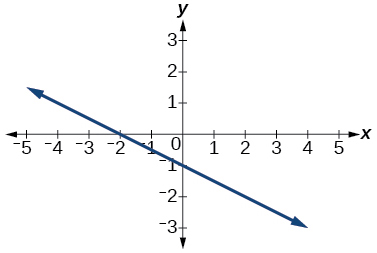35.36.37.38.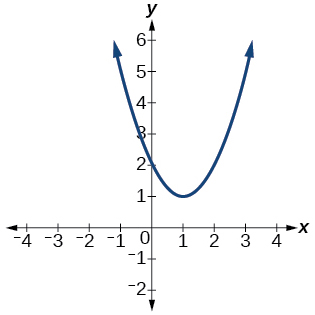For the following exercises, determine whether the graph of the function provided is a graph of a polynomial function. If so, determine the number of turning points and the least possible degree for the function.

39.Answer: Yes. Number of turning points is 2. Least possible degree is 3.

40.41.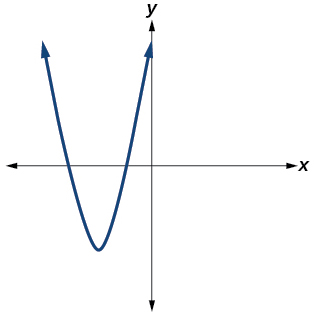Answer: Yes. Number of turning points is 1. Least possible degree is 2.

42.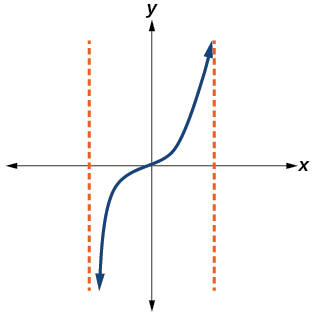43.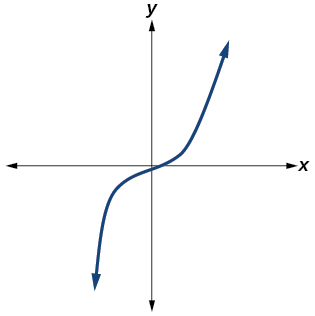Answer: Yes. Number of turning points is 0. Least possible degree is 3.

Exercise $$\PageIndex{44}$$No (the graph is not smooth)

45.Answer: Yes. Number of turning points is 0. Least possible degree is 1.

## Numeric

For the following exercises, make a table to confirm the end behavior of the function.

46. $$f(x)=−x^3$$

47. $$f(x)=x^4−5x^2$$

$$x$$ $$f(x)$$
10 9,500
100 99,950,000
–10 9,500
–100 99,950,000

as $$x→−∞,$$ $$f(x)→∞$$, as $$x→∞,$$ $$f(x)→∞$$

48. $$f(x)=x^2(1−x)^2$$

49. $$f(x)=(x−1)(x−2)(3−x)$$

$$x$$ $$f(x)$$
10 9,500
100 99,950,000
–10 9,500
–100 99,950,000

as $$x→−∞,$$ $$f(x)→∞$$, as $$x→∞,$$ $$f(x)→−∞$$

50. $$f(x)=\frac{x^5}{10}−x^4$$

## Technology

For the following exercises, graph the polynomial functions using a calculator. Based on the graph, determine the intercepts and the end behavior.

51. $$f(x)=x^3(x−2)$$The y-intercept is $$(0, 0)$$. The x-intercepts are $$(0, 0), (2, 0).$$ As $$x→−∞,$$ $$f(x)→∞$$, as $$x→∞,$$ $$f(x)→∞$$

52. $$f(x)=x(x−3)(x+3)$$

53. $$f(x)=x(14−2x)(10−2x)$$The y-intercept is $$(0,0)$$ . The x-intercepts are $$(0, 0), (5, 0), (7, 0)$$. As $$x→−∞$$, $$f(x)→−∞$$, as $$x→∞,$$ $$f(x)→∞$$

54. $$f(x)=x(14−2x)(10−2x)^2$$

55. $$f(x)=x^3−16x$$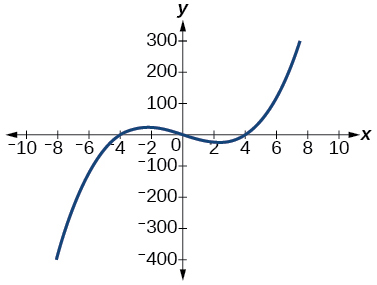The y-intercept is (0, 0). The x-intercept is $$(−4, 0), (0, 0), (4, 0)$$. As $$x→−∞$$, $$f(x)→−∞$$, as $$x→∞,$$ $$f(x)→∞$$

56. $$f(x)=x^3−27$$

57. $$f(x)=x^4−81$$The y-intercept is (0, −81). The x-intercept are $$(3, 0), (−3, 0)$$. As $$x→−∞,$$ $$f(x)→∞$$, as $$x→∞,$$ $$f(x)→∞$$

58. $$f(x)=−x^3+x^2+2x$$

59. $$f(x)=x^3−2x^2−15x$$The y-intercept is $$(0, 0)$$. The x-intercepts are $$(−3, 0), (0, 0), (5, 0).$$ As $$x→−∞$$, $$f(x)→−∞$$, as $$x→∞,$$ $$f(x)→∞$$

60. $$f(x)=x^3−0.01x$$

## Extensions

For the following exercises, use the information about the graph of a polynomial function to determine the function. Assume the leading coefficient is 1 or –1. There may be more than one correct answer.

61. The y-intercept is $$(0,−4)$$. The x-intercepts are $$(−2,0), (2,0)$$. Degree is 2.

End behavior: as $$x→−∞,$$ $$f(x)→∞$$, as $$x→∞,$$ $$f(x)→∞$$.

Answer: $$f(x)=x^2−4$$

62. The y-intercept is $$(0,9)$$. The x-intercepts are $$(−3,0), (3,0)$$. Degree is 2.

End behavior: as $$x→−∞,$$ $$f(x)→−∞$$, as $$x→∞,$$ $$f(x)→−∞$$.

63. The y-intercept is $$(0,0)$$. The x-intercepts are $$(0,0), (2,0)$$. Degree is 3.

End behavior: as $$x→−∞,$$ $$f(x)→−∞$$, as $$x→∞,$$ $$f(x)→∞$$.

Answer: $$f(x)=x^3−4x^2+4x$$

64. The y-intercept is $$(0,1)$$. The x-intercept is $$(1,0)$$. Degree is 3.

End behavior: as $$x→−∞$$, $$f(x)→∞$$, as $$x→∞$$, $$f(x)→−∞$$.

65. The y-intercept is $$(0,1)$$. There is no x-intercept. Degree is 4.

End behavior: as $$x→−∞,$$ $$f(x)→∞$$, as $$x→∞,$$ $$f(x)→∞$$.

Answer: $$f(x)=x^4+1$$

## Real-World Applications

For the following exercises, use the written statements to construct a polynomial function that represents the required information.

66. An oil slick is expanding as a circle. The radius of the circle is increasing at the rate of 20 meters per day. Express the area of the circle as a function of $$d$$, the number of days elapsed.

67. A cube has an edge of 3 feet. The edge is increasing at the rate of 2 feet per minute. Express the volume of the cube as a function of $$m$$, the number of minutes elapsed.

Answer: $$V(m)=8m^3+36m^2+54m+27$$

68. A rectangle has a length of 10 inches and a width of 6 inches. If the length is increased by $$x$$ inches and the width increased by twice that amount, express the area of the rectangle as a function of $$x$$.

Exercise $$\PageIndex{69}$$

An open box is to be constructed by cutting out square corners of $$x$$-inch sides from a piece of cardboard 8 inches by 8 inches and then folding up the sides. Express the volume of the box as a function of $$x$$.

$$V(x)=4x^3−32x^2+64x$$